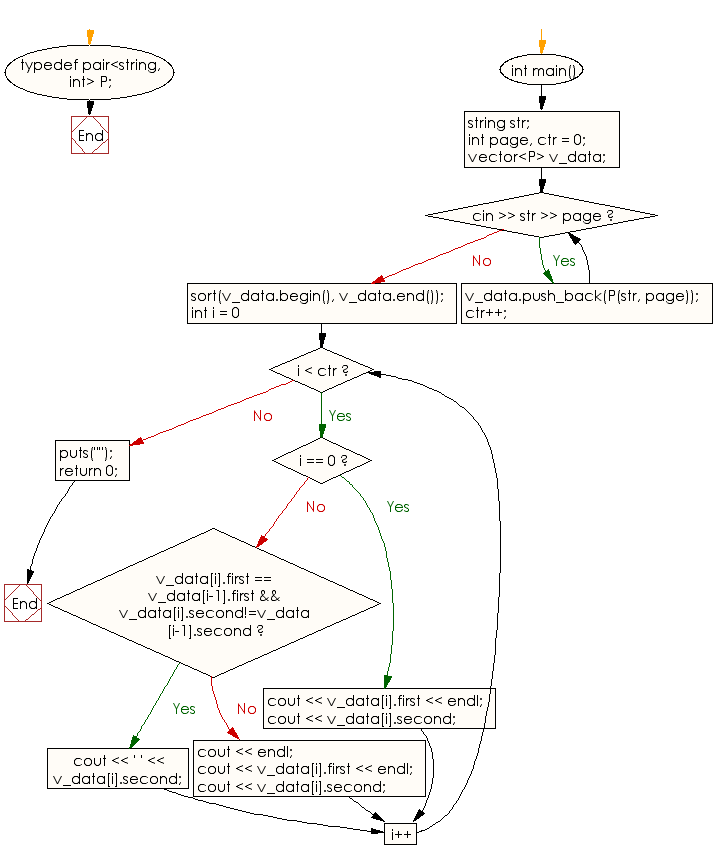﻿ C++ : Prints the word and corresponding page numbers

# C++ Exercises: Prints the word and a list of the corresponding page numbers

## C++ Basic: Exercise-82 with Solution

Write a C++ program that reads a list of pairs of a word and a page number, and prints the word and a list of the corresponding page numbers.

Sample Solution:

C++ Code :

``````#include <iostream>
using namespace std;
typedef pair<string, int> P;

int main()
{
string str;
int page, ctr = 0;
vector<P> v_data;

while (cin >> str >> page) {
v_data.push_back(P(str, page));
ctr++;
}

sort(v_data.begin(), v_data.end());
for (int i = 0; i < ctr; i++) {
if (i == 0) {
cout << v_data[i].first << endl;
cout << v_data[i].second;
continue;
}

if (v_data[i].first == v_data[i-1].first && v_data[i].second!=v_data[i-1].second) {
cout << ' ' << v_data[i].second;
continue;
} else {
cout << endl;
}
cout << v_data[i].first << endl;
cout << v_data[i].second;
}
puts("");
return 0;
}
``````

Sample Output:

```Sample Input: Python 2
HTML  4
CSS    3
Python 5
Python 3
HTML  2
CSS   6
Output:
CSS
3 6
HTML
2 4
Python
2 3 5
```

Flowchart:C++ Code Editor:

What is the difficulty level of this exercise?

﻿

## C++ Programming: Tips of the Day

Why is there no std::stou?

The most pat answer would be that the C library has no corresponding "strtou", and the C++11 string functions are all just thinly veiled wrappers around the C library functions: The std::sto* functions mirror strto*, and the std::to_string functions use sprintf.

Ref: https://bit.ly/3wtz2qA

We are closing our Disqus commenting system for some maintenanace issues. You may write to us at reach[at]yahoo[dot]com or visit us at Facebook